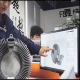# Three-Phase Circuit

A Three-Phase circuit is a circuit composed of a Three-Phase source, a three-phase load, and a three-phase transmission line. The most basic structural feature of this circuit is that it has one or more groups of power supplies. Each group of power supplies is composed of three sinusoidal power supplies with the same amplitude, the same frequency, and the same phase difference between each other, and the power supply and the load adopt a specific connection method. Three-phase circuits are widely used in power systems such as power generation, transmission, distribution, and high-power electrical equipment.

The three-phase power supply and the three-phase load have two connection modes: star and delta. When the three-phase power supply and the three-phase load are connected through the transmission line (the impedance is ZL) to form a three-phase circuit, five connection modes can be formed, respectively It is called Y0—Y0 connection (with center line), Y—Y connection (without center line), Y-△ connection, △-Y connection and △-△ connection, as shown in Figures 1 to 4, among which Figure 1 shows There are two midpoints, and the midpoints can be connected to the transmission line (the neutral line, whose impedance is ZN), which is called the three-phase four-wire system. In Figures 2 to 4, there are only three transmission lines, and there is no midline, which is called three-phase Three-wire system.

Symmetrical three-phase power supply
Symmetrical three-phase power supply is a power supply composed of 3 sinusoidal voltage sources with equal amplitude, same frequency, and initial phase difference of 120° connected to form a star (Y) or delta (△). These three power sources are called A-phase, B-phase and C-phase in turn.
The phase sequence (sequence) A, B, and C of the above-mentioned three-phase voltage is called positive sequence or sequence. On the contrary, it is called reverse order or reverse order. Power system generally adopts positive sequence.
Symmetrical three-phase voltage satisfies the conditions:
ua+ub+uc=0 or phasor expression
The symmetrical three-phase voltage is provided by a three-phase generator.

Asymmetric circuit
In a three-phase circuit, as long as there is a part of the asymmetry, it is called an asymmetric three-phase circuit.
In a three-phase circuit, the complex power absorbed by the three-phase load is equal to the sum of the various complex powers.
The instantaneous power of a three-phase circuit is the sum of the instantaneous power of each phase load.
In a three-phase three-wire circuit, whether symmetrical or not, two power meters can be used to measure three-phase power. That is the two-watt notation.

Features
A symmetrical three-phase power supply is connected to a symmetrical three-phase load, which is called a symmetrical three-phase circuit (in general, the power supply is always symmetrical). There are Y-Y, △-Y, △-△, Y-△ connection modes between the three-phase power supply and the load. The three-phase circuit is actually a special type of sinusoidal AC circuit. In a three-phase circuit, the connection method of a three-phase load is determined by the rated voltage of each phase of the load and the line voltage of the power supply. Because the response of each group in a symmetrical three-phase circuit is a symmetrical quantity with the same phase sequence as the excitation. Therefore, not only the effective value of the phase voltage but also the effective value of the phase current is equal for each phase. Moreover, the phase difference between the voltage and current of each phase is also equal. Thus the active power of each phase is equal.Author: Yoyokuo